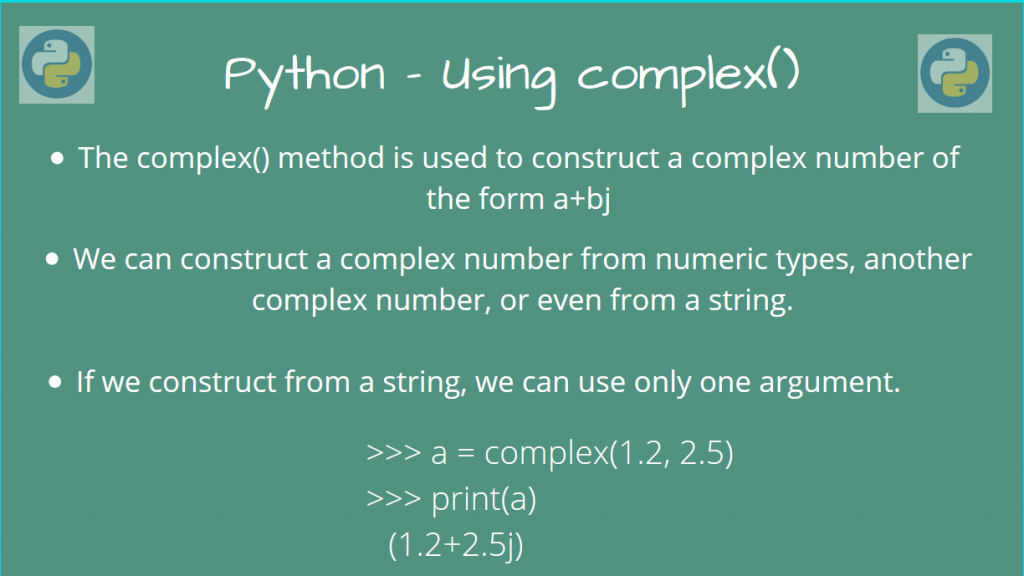# How to Use the Python complex() methodThe Python complex() method is used to create a complex number from other primitive data-types. This is useful whenever you want to perform complex arithmetic and transformations quickly.

Let’s take a look at how we can use this method.

## Syntax of Python complex()

This function returns a complex number, which contains a real part, and an imaginary part. The complex number is of the `class complex` datatype.

Therefore, the function call syntax is:

```class complex([real[, imag]])
```

The complex number will be of the form:

```complex_number = real + imag*j
```

Here, `j` is the imaginary number (sqrt(-1))

The Python `complex()` method will return this complex number.

Let’s look at some examples, to get familiar with this method.

You may think that we could use only integers and floats to construct the complex number, but that’s not that case!

We can also construct it from a string, hexadecimal, binary too, or even from another complex number!

We’ll take a look at some examples, to illustrate this point.

## Using the Python complex() method

### Calling complex() without any parameter

We can call this method without passing any arguments to it. This will return the zero complex number `0j`.

```a = complex()
print(type(a))
print(a)
```

Output

```<class 'complex'>
0j
```

### Calling Python complex() using numeric parameters

This will construct the desired complex number of the form `a + bj`, where `a` and `b` are the numeric parameters.

```a = complex(1, 2)
print(a)

b = complex(1, 1.5)
print(b)

c = complex(-1.5, 3.414)
print(c)
```

Output

```(1+2j)
(1+1.5j)
(-1.5+3.414j)
```

### Calling complex() with Hexadecimal / Binary arguments

We can also directly pass hexadecimal or binary number into this, without converting it to an integer.

```a = complex(0xFF)  # hexadecimal
print(a)

b = complex(0b1010, -1)  # binary
print(b)
```

Output

```(255+0j)
(10-1j)
```

### Calling complex() using another complex number

We can also pass another complex number, when constructing a complex number using `complex()`

```a = 1 + 2j
b = complex(a, -4) # Construct using another complex number
print(b)
c = complex(1+2j, 1+2J)
print(c)
```

Output

```(1-2j)
(-1+3j)
```

Since both the arguments are added according to the form `a + b*j`, in the first case, the result will be: 1 + 2j + (-4*j) = 1 – 2j

In the second case, we have (since j*j = -1): 1+2j + (1+2j)*j = (1 + 2j + j – 2) = -1+3j

### Calling Python complex() using string arguments

We can also pass string with it, provided that it does not have any white space in between. The string must only be of the form “a+bj”, i.e, a string which can represent a complex number.

If we are using a string, we can only use one argument.

```a = complex("1+2j")
print(a)
```

Output

```(1+2j)
```

If the string has whitespaces or any other extraneous character, a `ValueError` exception will be raised.

```b = complex("2 + 4j")
print(b)
```

This will raise a `ValueError`, since there are spaces in the string.

Similarly, if we pass two arguments to Python `complex()`, it will raise a `TypeError` exception, as we are not allowed to pass multiple arguments if the first argument is a String.

## Conclusion

In this article, we learned how we can construct a complex number from different data-types, using the built-in `complex()` method. If you want to learn about other Python built-in functions visit here.